# SBI PO Prelims Quantitative Aptitude Questions 2019 – Day 18

SBI PO 2019 Notification will be expected soon. It is one of the most expected recruitment among the banking aspirants. Every year the exam pattern for SBI PO has been changing. Depends upon the changing of exam pattern the questions are quite harder compare to the previous year. So the questions are in high level than the candidate’s assumption.

As per the latest trend, our IBPS Guide is providing the updated New Exam Pattern Quantitative Aptitude questions for SBI PO 2019 Day 17. Our Skilled experts were mounting the questions based on the aspirant’s needs. So candidates shall start your preparation and practice on daily basis with our SBI PO pattern quantitative aptitude questions 2019 day 18. Start your effective preparation from the right beginning to get success in upcoming SBI PO 2019.

[WpProQuiz 5327]

### Click Here for SBI PO Pre 2019 High-Quality Mocks Exactly on SBI Standard

1) A bus covers the first _____ Km in 25 mins and the remaining 87 Km in one hour. Then the average speed of the bus is _____ Km/hr.

Which of the following option satisfies the given condition?

a) 32, 72

b) 44, 100

c) 32, 84

d) 36, 84

e) None of these

2) The compound interest on a certain sum for _____ year/years at 10% per annum is Rs. _____. Then the simple interest for the same sum on 2 years at 10 % per annum is Rs. 6000.

Which of the following option satisfies the given condition?

I) 2, 6300

II) 3, 9930

III) 1, 3000

a) Only I and II

b) Only II and III

c) Only I and III

d) Only II

e) All I, II and III

3) The speed of the man in still water is ______ km/hr and the rate of flow of water is 7.5 km/hr. He takes 30 hours to row to a place and come back. The distance between these two places is _______.

Which of the following option satisfies the given condition?

a) 14.5, 170

b) 12.5, 120

c) 10.5, 90

d) 13.5, 140

e) Both b and d

4) A, B and C entered into a partnership with investment in the ratio of      _______ respectively. After one year, B doubled his investment. At the end of two years, they earned a profit of Rs. ______. The share of B in the profit is Rs. 30000.

Which of the following option satisfies the given condition?

I) 3: 5: 12, 90000

II) 3: 6: 7, 65000

III) 4: 5: 8, 78000

a) Only I and II

b) Only II and III

c) Only I and III

d) Only II

e) All I, II and III

5) A person covers ____ km in 6 hours. He covers some distance by bus at the average speed of 50 km/hr and the remaining distance by train at the average speed 90 km/hr. The distance covered by the train is _____ Km.

a) 480, 360

b) 420, 240

c) 360, 90

d) 450, 280

e) Both a and c

Directions (Q. 6 – 10): Study the following information carefully and answer the given questions:

The following table shows total number of people going for a movie in a different Cinema theatre and percentage of male among them and the ratio of total number of people seeing English and Hindi movie in the theatre.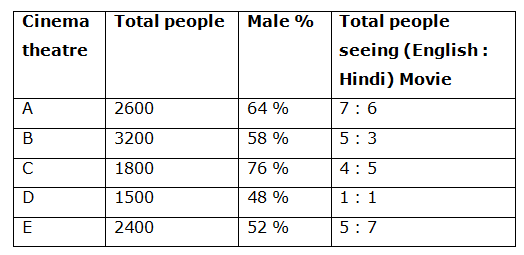Note: Only two language movies English and Hindi running in the theatres.

6) Total number of female going for a movie in theatre C is approximately what percentage of total number of male going for a movie in theatre A?

a) 26 %

b) 35 %

c) 44 %

d) 58 %

e) 65 %

7) Find the ratio between the total number people seeing English movie in theatre E to that of total number of people seeing Hindi movie in theatre C?

a) 2: 5

b) 3: 4

c) 1: 1

d) 5: 7

e) None of these

8) Find the difference between the total number of female going for a movie in theatre B and D together to that of total number of people going for a movie in theatre E?

a) 354

b) 276

c) 390

d) 428

e) None of these

9) Find the total number of people seeing English movie in theatre B, C and D together?

a) 3780

b) 3060

c) 3890

d) 3550

e) None of these

10) Total number of people going for a movie in theatre E is approximately what percentage more than total number of people going for a movie in theatre C?

a) 33 %

b) 47 %

c) 50 %

d) 66 %

e) 25 %

Option (a)

Average speed = Total distance ÷ Total time

Total time = (25/60) + 1 = (17/12) hours

Total distance = 32 + 87 = 119 km

Average speed = 119/(17/12)

= > 84 km/hr ≠ 72 km/hr

This doesn’t satisfy the given condition.

Option (b)

Average speed = Total distance ÷ Total time

Total time = (25/60) + 1 = (17/12) hours

Total distance = 44 + 87 = 131 km

Average speed = 131/(17/12)

= > 92.47 km/hr ≠ 100 km/hr

This doesn’t satisfy the given condition.

Option (c)

Average speed = Total distance ÷ Total time

Total time = (25/60) + 1 = (17/12) hours

Total distance = 32 + 87 = 119 km

Average speed = 119/(17/12)

= > 84 km/hr

This satisfies the given condition.

Option (d)

Average speed = Total distance ÷ Total time

Total time = (25/60) + 1 = (17/12) hours

Total distance = 36 + 87 = 123 km

Average speed = 123/(17/12)

= > 86.82 km/hr ≠ 84 km/hr

This doesn’t satisfy the given condition.

Principle amount = (6000*100)/(10*2) = Rs. 30000

Option (I)

CI = 30000*(110/100)*(110/100) – 30000

= > 36300 – 30000 = Rs. 6300

This satisfies the given condition.

Option (II)

CI = 30000*(110/100)*(110/100)*(110/100) – 30000

= > 39930 – 30000 = Rs. 9930

This satisfies the given condition.

Option (III)

CI = 30000*(110/100) – 30000

= > 33000 – 30000 = Rs. 3000

This satisfies the given condition.

Option (a)

Speed of man in still water = 14.5 km/hr

Speed of stream = 7.5 km/hr

Speed of upstream = 14.5 – 7.5 = 7 km/hr

Speed of downstream = 14.5 + 7.5 = 22 km/hr

According to the question,

(x/22) + (x/7) = 30

29x/(22*7) = 30

X = 159.31 km

This doesn’t satisfy the given condition.

Option (b)

Speed of man in still water = 12.5 km/hr

Speed of stream = 7.5 km/hr

Speed of upstream = 12.5 – 7.5 = 5 km/hr

Speed of downstream = 12.5 + 7.5 = 20 km/hr

According to the question,

(x/20) + (x/5) = 30

5x/20 = 30

X = 120 km

This satisfies the given condition.

Option (c)

Speed of man in still water = 10.5 km/hr

Speed of stream = 7.5 km/hr

Speed of upstream = 10.5 – 7.5 = 2.5 (or) 5/2 km/hr

Speed of downstream = 10.5 + 7.5 = 18 km/hr

According to the question,

(x/18) + (2x/5) = 30

41x/(18*5) = 30

X = 65.85 km

This doesn’t satisfy the given condition.

Option (d)

Speed of man in still water = 13.5 km/hr

Speed of stream = 7.5 km/hr

Speed of upstream = 13.5 – 7.5 = 6 km/hr

Speed of downstream = 13.5 + 7.5 = 21 km/hr

According to the question,

(x/21) + (x/6) = 30

27x/(21*6) = 30

X = 140 km

This satisfies the given condition.

Option (I)

The share of A, B and C,

= > [3*2] : [5*1 + 10*1] : [12*2]

= > 6 : 15 : 24 = 2 : 5 : 8

5’s = 30000

1’s = 6000

Total profit = 15’s = Rs. 90000

This satisfies the given condition.

Option (II)

The share of A, B and C,

= > [3*2] : [6*1 + 12*1] : [7*2]

= > 6 : 18 : 14 = 3 : 9 : 7

9’s = 30000

1’s = 30000/9

Total profit = 19’s = (30000/9)*19 = Rs. 63333.33

This doesn’t satisfy the given condition.

Option (III)

The share of A, B and C,

= > [4*2] : [5*1 + 10*1] : [8*2]

= > 8: 15: 16

15’s = 30000

1’s = 2000

Total profit = 39’s = Rs. 78000

This satisfies the given condition.

Option (a)

Average speed of the person= 480/6 = 80 km/hr

By applying the rule of allegation we get,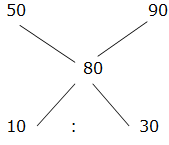= > 1: 3

Ratio between distances covered as 1: 3

So, Distance covered by train= 480*3/4 = 360 km

This satisfies the given condition.

Option (b)

Average speed of the person= 420/6 = 70 km/hr

By applying the rule of allegation we get,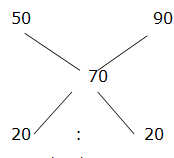= > 1 : 1

Ratio between distances covered as 1 : 1

So, Distance covered by train= 420*1/2 = 210 km

This doesn’t satisfy the given condition.

Option (c)

Average speed of the person= 360/6 = 60 km/hr

By applying the rule of allegation we get,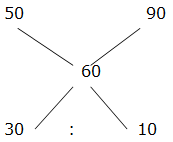= > 3: 1

Ratio between distances covered as 3: 1

So, Distance covered by train= 360*1/4 = 90 km

This satisfies the given condition.

Option (d)

Average speed of the person= 450/6 = 75 km/hr

By applying the rule of allegation we get,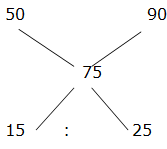= > 3: 5

Ratio between distances covered as 3: 5

So, Distance covered by train= 450*5/8 = 281.25 km

This doesn’t satisfy the given condition.

Direction (6-10) :

Total number of female going for a movie in theatre C

= > 1800*(24/100) = 432

Total number of male going for a movie in theatre A

= > 2600*(64/100) = 1664

Required % = (432/1664)*100 = 26 %

The total number people seeing English movie in theatre E

= > 2400*(5/12) = 1000

The total number of people seeing Hindi movie in theatre C

= > 1800*(5/9) = 1000

Required ratio = 1000: 1000 = 1: 1

The total number of female going for a movie in theatre B and D together

= > 3200*(42/100) + 1500*(52/100)

= > 1344 + 780 = 2124

The total number of people going for a movie in theatre E = 2400

Required difference = 2400 – 2124 = 276

The total number of people seeing English movie in theatre B, C and D together

= > 3200*(5/8) + 1800*(4/9) + 1500*(1/2)

= > 2000 + 800 + 750 = 3550